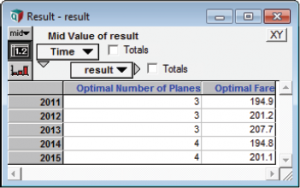# Module 7: Embedding an NLP in a Dynamic Loop

The solution in Module 6 advises the airline to decrease the number of planes in service between 2014 and 2015. What if the airline has a lease agreement that does not allow them to return planes? In this case we would like to impose a constraint that requires the Number of Planes to increase or stay the same from year to year.

The lower bound of the constraint depends on Number of Planes in the previous year. The constraint is based on a dynamic quantity. You can embed an NLP inside a dynamic loop to impose dynamic constraints. There is only one restriction:

• Bounds on Decision variables cannot be recursively dependent on Time (or other index on which the Dynamic loop is based). To impose recursively time-dependent bounds, they must be entered as constraints instead of Decision bounds attributes.

Let’s try it! Starting with Module 6, create a new variable titled Previous Number of Planes. (We will have to use a dynamic definition since we are referring to a previous time step.) Then create the constraint based on the new variable.

Variable Previous_Planes := Dynamic(0, Number_of_Planes[Time - 1])
Constraint No_Plane_Decrease := Number_of_Planes >= Previous_Planes
Variable Opt := DefineOptimization(
Decisions: Number_of_Planes, Fare,
Constraints: No_Plane_Decrease
Maximize: NPV_Profit)The dynamic constraint is satisfied.